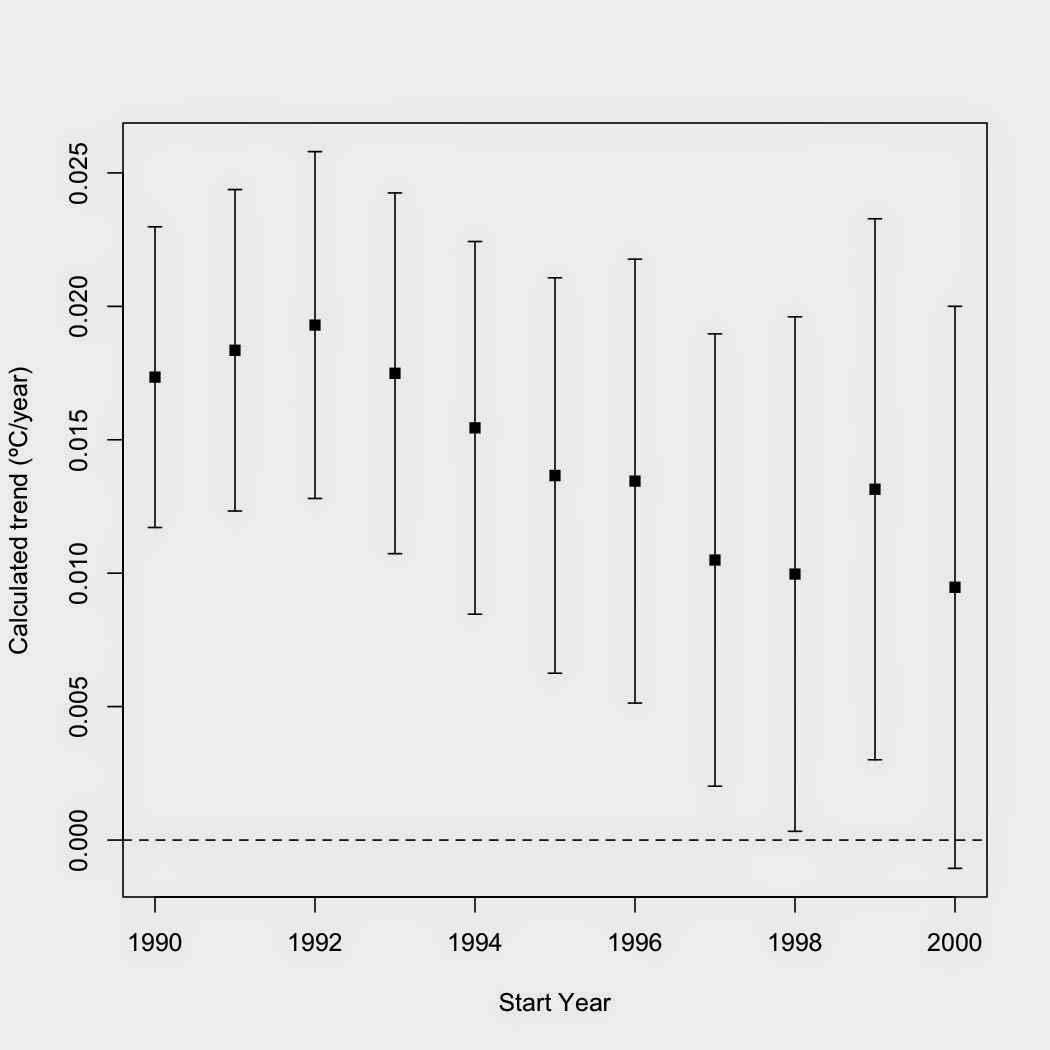### The "no warming" claim rises from the dead yet again.

Like a movie vampire, this one keeps coming back no matter how many stakes are driven through its heart.  I've covered this one (here, here, and here).  Bluntly: There is absolutely no evidence that global warming has stopped.  For global warming to stop, the Earth's energy balance must be either zero or negative.  The most recent estimates for the energy imbalance are generally between +0.5 W/m2 and +1.0 W/m2.  The only way the Earth is not going to warm while it is gaining energy is if the laws of thermodynamics magically do not apply.  If the Earth is gaining energy, some part of it, somewhere, must be getting warmer.  The heat must go into either melting ice, warming the oceans, warming the land, or warming the atmosphere (or some combination thereof).

Ah, but what about the atmosphere?  Has warming in the atmosphere stopped?  Again, a blunt "No."  Almost every claim of "no warming" comes from someone trying to start a linear trend line at 1998—an outlier year which saw the strongest El Niño event on record.  How do we know 1998 was an outlier?  Simple statistics.

First, I took coverage-corrected HadCRUT4 monthly temperature data between January 1970 and December 2013 and calculated the yearly average.  I then fitted a loess regression to that data.  The result?

I then plotted the residuals for that plot.  For those who are not statisticians, residuals are calculated by subtracting the predicted value based on the trend from each data point.  Residuals show how far each data point deviates from the trend, with a point right on the trend coming in with a residual of zero.  Taking the residuals also removes the trend from the data, which is handy for analyses for cycles and the like that occur around the trend.

Note that bright red point?  That's 1998.  Notice that it deviates farther from the trend than any of the points after it?  That's important as it essentially tells us that if we start a linear trend from 1998, that single data point will influence the trend more than any other year from 1999 to 2013.  Now I can hear someone saying, "But that's with a fancy-schmancy nonlinear regression.  What does plain old linear regression show?"

The residual plot reveals the following:

It doesn't really matter which regression technique you use.  1998 was an outlier by any definition.  How does starting a linear regression near 1998 affect the trend?  Quite a bit.  First, as the start point gets closer to 1998, the beginning of the trend line gets "pulled up" toward the 1998 outlier, thereby decreasing the apparent slope of the line.  Second, you have fewer and fewer data points left in the analysis to compensate for that upward pull, making the 1998 outlier more influential on the trend as the dataset gets smaller.  Third, your analysis loses power as the number of data points decrease and becomes less likely to show that a trend is statistically significant, even when trends actually exist.  You can easily see the affect of starting closer and closer to the 1998 outlier in the graph below:

If I plot the calculated trends versus the year each trend starts, I get the following:Trends versus start year.  Error bars are the 95% confidence intervals.
First, the calculated trend gets lower as one gets closer to 1998 (closer to the outlier) and the confidence intervals around the trend get larger as less data is used in each calculated trend.  Second, all the confidence intervals overlap—there is no point as yet where one can safely say that the trend has actually changed.  Third—and somewhat surprising—even the warming trend since 1998 is statistically significant.  Guess deniers will have to find another start point for their "no warming since..." claims.

In short, all a claim of "no warming" shows is that the person making it either a) doesn't understand how global warming works and/or b) doesn't understand statistics.  For many such individuals, I'd guess that the real reason is both a and b.